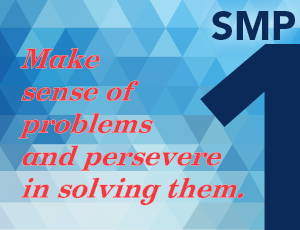<img height="1" width="1" alt="" style="display:none" src="https://www.facebook.com/tr?id=940171109376247&amp;ev=PageView&amp;noscript=1">

# Dedicated to TeachersBy Sue O’Connell (@SueOConnellMath), author of Putting the Practices Into Action.

It seems ironic that the first Standard for Mathematical Practice focuses on problem solving. When I was a student, math problem solving seemed to be an afterthought. Problems were at the bottom of the textbook page or at the end of a lesson. My perception was that math was about computation. Most of my time was spent memorizing facts and algorithms and solving for the right answer. Thank goodness, then, that we have revised our goals for today’s students. While I do hope they can find the answers to math computations, I know they can accomplish so much more. With guidance from the Common Core Standards for Mathematical Practice, we focus on ways to help our students develop the skills needed to be proficient mathematicians who solve problems.But how do you teach someone to be a problem solver? That is a particular challenge for most teachers today who, like me, were assigned problems to solve but not taught to be problem solvers by their own teachers. What can we do differently for our students? As we explore the expectations in Practice Standard #1, we gain insights into the complexity of the task. Are we considering process, strategies, and attitudes as we explore ways to teach our students to solve problems?

• Process: Do students understand the process for solving problems? What do they do first? Next? How should they approach and move through a problem-solving task?
• Strategies: Do students have a repertoire of strategies to solve different types of problems? Do they understand when to add, subtract, multiply, and divide? Do they have other strategies to rely on like drawing diagrams or organizing data to look for patterns that might lead them to solutions? Are they able to choose and adjust strategies as needed?
• Attitudes: Do they believe they can solve problems? Do they persevere when problems are complex? Do they have patience when a first approach doesn’t work?

While the teaching of problem solving is complex, there are many steps we can take to foster our students’ problem-solving skills.

1. Integrate problem solving with computations. Begin lessons with a problem-solving task. Whether students are adding single-digit numbers, subtracting multi-digit numbers, multiplying fractions, or dividing decimals, the problem context helps students make sense of the computations that follow. The problem gives a context for the operations and helps students better understand when and why we use each operation. In this way, we are simultaneously teaching computations through problems and giving our students experience solving problems.
2. Start with comprehension. Focus on first understanding the problem, then solving it. Ask students to retell the problem. Do they understand what is happening? Can they tell you the critical data? Can they justify why a particular operation would make sense for solving this problem?
3. Encourage students to visualize the problem. One part of comprehension is the ability to visualize the situation. Can students draw a diagram or show the problem using manipulatives?
4. Focus on building a strong understanding of operations. Emphasize the action of operations, not the key words. Are we comparing quantities? Are we splitting a total into groups? Are we combining quantities to find a total? Students who understand the action of each operation, through experiences with many contexts that show that action, are better able to select the operation—and build the equation—that makes sense for a given problem.
5. Ask deep questions. Ask students to defend their choice of operations. Ask students to justify the data they choose to use or not use. Ask students to find other ways to solve the problem or to critique the methods of others. Ask students to explain their methods and prove the reasonableness of their answers. Pose questions that prompt students to talk about their thinking.
6. Get students talking to one another. Set up opportunities for students to discuss the problem, from the initial interpretation of the task through to the final presentation of their method and solution. Have students debate their methods for both accuracy and efficiency.
7. Pose problems that encourage perseverance. Pose problems with multiple steps or with multiple answers. Discuss ways students may have gotten “stuck” when solving a problem and how they got “unstuck.” Praise patience, reflection, and perseverance.

Problem solving is the starting point and ending point in meaningful math instruction. By beginning with problems, we set a context for the math skills students learn, and as students are able to apply varied math skills to solve problems they demonstrate their ability to use and apply mathematics. As our students make sense of problems and persevere in solving them, they are doing the work of a mathematician.

♦ ♦ ♦ ♦Sue O’Connell is a nationally known speaker and education consultant who directs Quality Teacher Development. She is the coauthor of Putting the Practices Into Action and the Mastering the Basic Math Facts series. Her newest curricular resource, Math in Practice, will be available in early summer. Follow her on Twitter @SueOConnellMath.